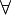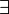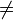# 12-240/Classnotes for Thursday September 13

In the second day of the class, the professor continues on the definition of a field.

## Definition of a field

Combined with a part from the first class, we have a complete definition as follow:

A field is a set "F' with two binary operations +,x defind on it, and two special elements 0 ≠ 1 such that

F1: commutative law$\forall \!\,$ a, b$\in \!\,$ F: a+b=b+a and a.b=b.a

F2: associative law$\forall \!\,$ a, b, c$\in \!\,$ F: (a+b)+c=a+(b+c) and (a.b).c= a.(b.c)

F3: the existence of identity elements$\forall \!\,$ a$\in \!\,$, a+0=a and a.1=a

F4: existence of inverses$\forall \!\,$ a$\in \!\,$ F \0,$\exists \!\,$ c, d$\in \!\$ F such that a+c=o and a.d=1

F5: contributive law$\forall \!\,$ a, b, c$\in \!\,$ F, a.(b+c)=a.b + a.c

## Theorems

Theorem 1: Cancellation laws$\forall \!\,$ a, b, c$\in \!\,$ F

if a+c=b+c, then a=b

if a.c=b.c and c$\ne \!\,$0, then a=b

Theorem 2: Identity uniqueness

Identity elements 0 and 1 mentioned in F3 are unique$\forall \!\,$ a, b, b'$\in \!\,$ F

if a+b=a and a+b'=a, then b=b'=0

if a.b=a and a.b'=a and a$\ne\!\,$0, then b=b'=1

Theorem 3: Inverse uniqueness

Elements c and d mentioned in F4 are unique$\forall \!\,$ a, b, b'$\in \!\,$ F

if a+b=0 and a+b'=0, then b=b'

if a.b=1 and a.b'=1, then b=b'

Theorem 4$\forall \!\,$ a$\in \!\,$ F

-( -a) = a

Theorem 4$\forall \!\,$ a$\in \!\,$ F

0.a= 0

## Significance

inverse uniqueness

It makes sense to define an operation -: F -> F called "negation"

For a$\in\!\,$ F define -a to be equal that b$\in\!\,$ F for which a+b=0, i.e, a+(-a)=0

Ex: F(5)={0,1,2,3,4}, define +,x

Question 1: What is(-3)?

Answer: -3=2 and -3 is unique

Similarly, the inverse uniqueness also makes sense a^(-1)

identity uniqueness